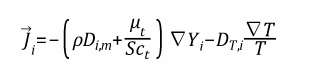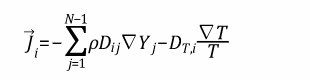## Fluids

•Dino
Subscriber
Hello,nI am writing a UDF and trying to call the molecular diffusion coefficient of species i. In the UDF manual, I see only C_DIFF_L(c, t, i, j) and it is defined as the laminar species diffusivity. So, C_DIFF_L(c, t, i, j) is the binary diffusion coefficient of species i in species j?nBut what I want is the molecular diffusion coefficient of species i, can I call it through this C_DIFF_L(c, t, i)? or do I need to write a UDF to calculate it since it is a function of the mass fraction of species i and the binary diffusion coefficient?nPlease help.nThanks,n
•wcao
Ansys Employee
Hello,nYou are correct that the C_DIFF_L(c, t, i, j) is the binary diffusivity. If you would like to have D_i,m, you cann1 Calculate it by subtracting the turbulent part from the C_DIFF_EFF(c,t,i) via the equation;n2 Calculate by the summing up D_ij along j:nThank you.n